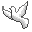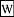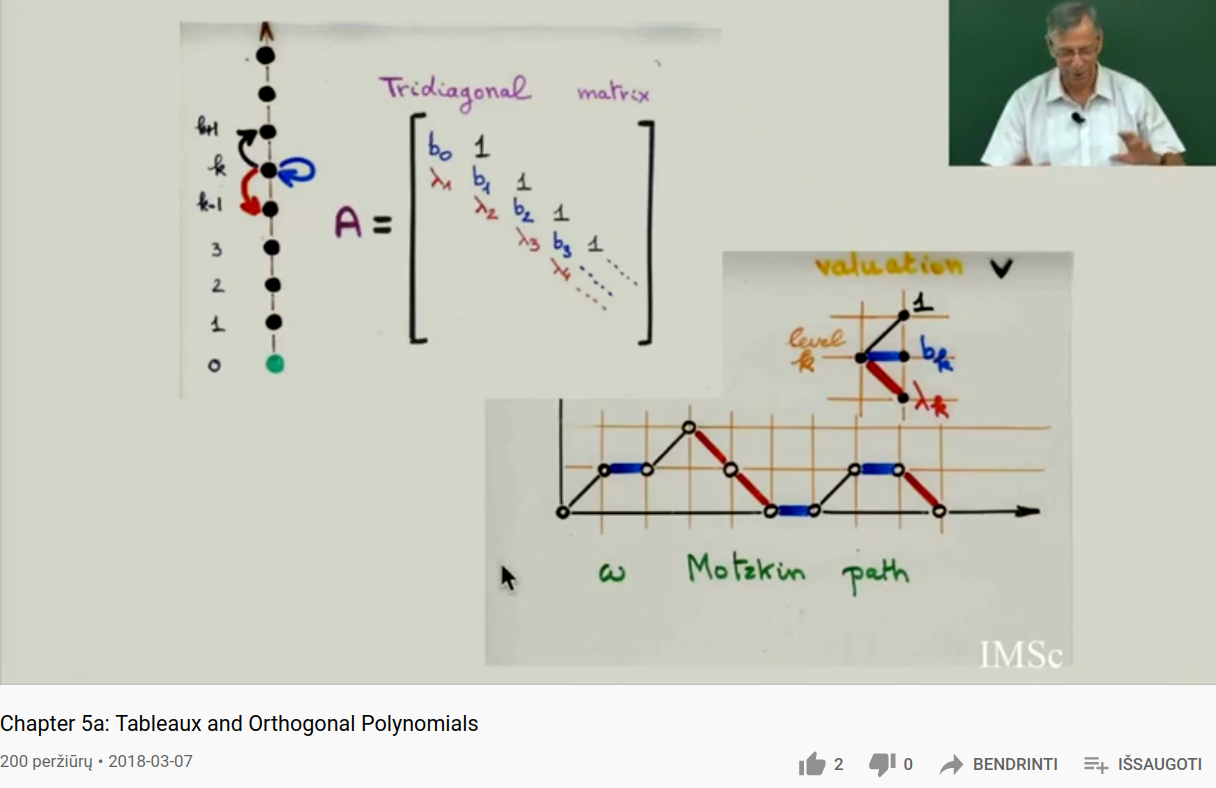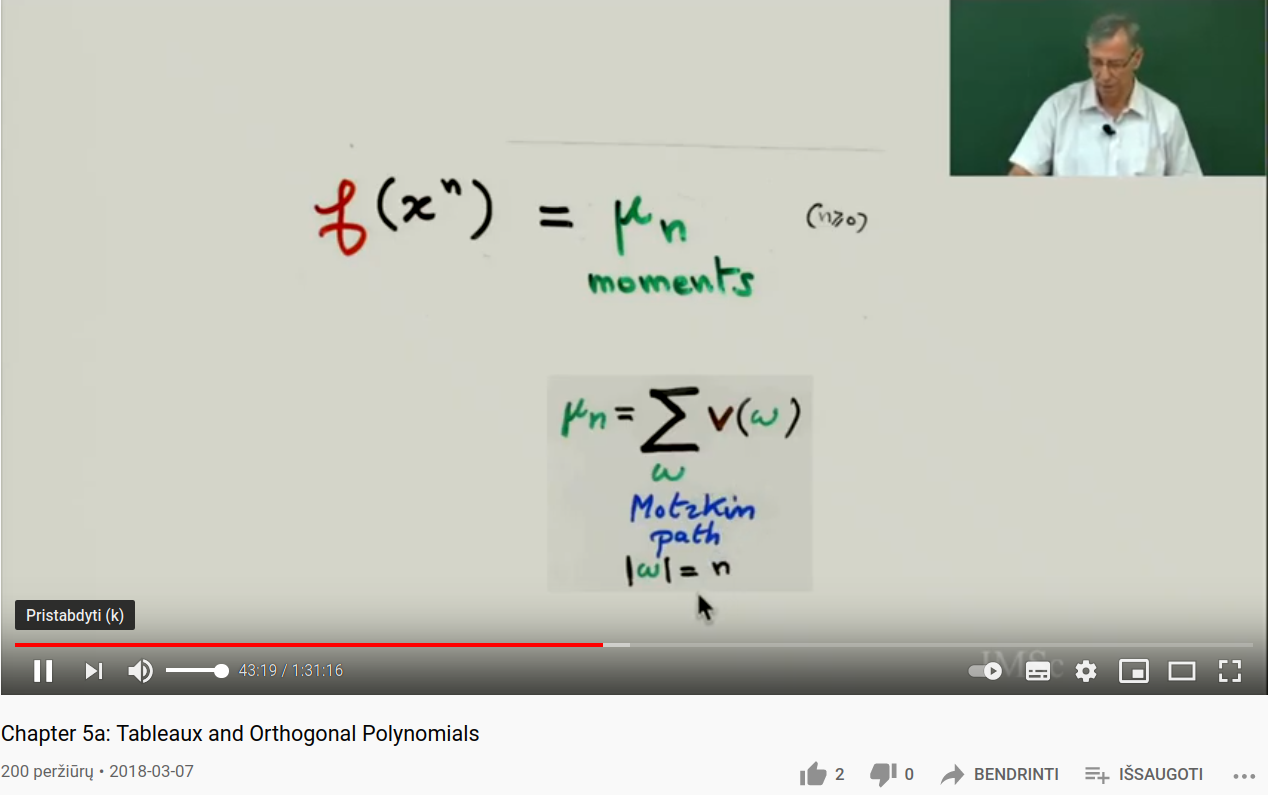Community

Contact

• Andrius Kulikauskas
• m a t h 4 w i s d o m @
• g m a i l . c o m
• +370 607 27 665
• Eičiūnų km, Alytaus raj, LithuaniaMy original work is in the Public Domain for you to use in your best judgement.

• Patreon
• Paypal to ms@ms.lt
•BookshelfAs an Amazon Associate I earn from qualifying purchases.

Thank you!

Weights

 Polynomial Weight {$w(x)$} {$\rho (x)$} Hermite {$e^{-x^2}$} {$1$} Charlier step function {$\frac{a^k}{k!}$} on interval {$[k,k+1)$} Laguerre {$x^\alpha e^{-x}$} {$x$} Meixner step function {$\frac{a^k(\beta)_k}{k!}$} on interval {$[k,k+1)$} Meixner-Pollaczek {$\frac{|\Gamma(\frac{n+ix}{2})|^2}{|\Gamma(\frac{n}{2})|^2}e^{-x\textrm{tan}^{-1}\delta}$} Jacobi {$(1-x)^{\alpha}(1+x)^\beta$} {$1-x^2$}

For a positive even integer {$n=2N$} we have

{$$\frac{|\Gamma(\frac{n+ix}{2})|^2}{|\Gamma(\frac{n}{2})|^2}e^{-x\textrm{tan}^{-1}\delta} = \frac{\pi}{2}x \;\textrm{csch}(\frac{\pi x}{2}) \prod_{k=1}^{N-1}(1+\frac{x^2}{4k^2})e^{-x\textrm{tan}^{-1}\delta}$$}

and for a positive odd integer {$n=2N+1$} we have

{$$\frac{|\Gamma(\frac{n+ix}{2})|^2}{|\Gamma(\frac{n}{2})|^2}e^{-x\textrm{tan}^{-1}\delta} = \textrm{sech}\;(\frac{\pi x}{2})\prod_{k=1}^{N}(1+\frac{x^2}{(2k-1)^2})e^{-x\textrm{tan}^{-1}\delta}$$}

Moments

The {$k$}th moment {$\mu_k$} of an orthogonal polynomial is gotten by integrating {$x^k$} against the weight {$\textrm{dw}$}.

{$$\mu_k=\int x^k \textrm{dw}$$}

In the case of the various Sheffer polynomials, these moments are fundamental numbers in combinatorics which count the most basic objects.

 Hermite (n-1)!! or 0 permutations of n where each cycle is a pair Charlier Bell number {$B_n$} set partitions of n (equivalence relations) Laguerre n! permutations of n (strict orderings) Meixner-Pollaczek Secant number {$A_{2n}$} alternating permutations of 2n Meixner ordered Bell number ordered set partitions of n (weak orderings)

I need to check the secant numbers... A relevant paper is P.Njionou Sadjang, W.Koepf, M.Foupouagnignia. On moments of classical orthogonal polynomials. 2015. I'm also curious about the Jacobi polynomials and other polynomials.

TheBell numbers also count permutations that avoid patterns of the form 1-23.

Other non-Sheffer polynomials have interesting moments:

 Chebyshev I {$\binom{2n}{n}$} ways of choosing half from {$2n$} Chebyshev II Catalan {$C_n = \frac{1}{n+1}\binom{2n}{n}$} {$n$} well formed parentheses

TheHankel determinants of the Catalan numbers are always equal to {$1$}.

Permutations

Zeng has an interpretation of the moment

{$$L(x^n)=\sum_{\sigma\in S_n}u_1^{ad\sigma} u_2^{da\sigma} u_3^{aa\sigma} u_4^{dd\sigma} a_1^{fix\sigma} b_1^{cycle\sigma}$$}

Here {$ad\sigma$} means number of ascents-descents, {$da\sigma$} – descents-ascents, {$aa\sigma$} – ascents-ascents, {$dd\sigma$} descents-descents, {$fix \sigma$} – fixed points, and {$cycles \sigma$} – cycles.

For us we have {$k=u_1u_2$} but also {$k=(-\alpha)(-\beta)$}. And we have {$l=u_3+u_4$} but also {$l=(-\alpha)+(-\beta)$}. Note that the variables in the equation above are simply placeholders. So we can choose to set {$u_1=-\alpha$}, {$u_2=-\beta$}, {$u_3=-\alpha$}, {$u_4=-\beta$}. We also note that descents {$d\sigma=da\sigma + dd\sigma$} and ascents {$a\sigma=aa\sigma + ad\sigma$}. And we can also set {$a_1$}=0, which means that we have no fixed points in our permutations, which means they are all derangements. And we have {$c=(b_1-1)k$}, which means {$b_1=\frac{k+c}{k}=\frac{-\gamma}{\alpha\beta}$}. Thus we have:

{$$L(x^n)=\sum_{\sigma\in S_n}(-\alpha)^{a\sigma} (-\beta)^{d\sigma} 0^{fix\sigma}(\frac{-\gamma}{\alpha\beta})^{cycle\sigma}$$}

Motzkin paths

Consider the recurrence relation {$P_{n+1}(x)=(x-b_n)P_n(x)-\lambda_nP_{n-1}(x)$}.

The moments {$\mu_n$} are given by the sum of the weights {$1$}, {$b_k$}, {$\lambda_k$} on the Motzkin path length {$n$}. Here {$k$} is the altitude of the path at a particular point. Each step along the path is given by the application of the tridiagonal matrix. The Motzkin path of length {$n$} arises from multiplying the matrix {$n$} times and considering those paths that "do nothing" in that they start and end at {$k=0$}.

Note that for Sheffer polynomials we furthermore have that {$b_k=ak+b$} and {$\lambda_k=k(ck+d)$} where {$c\geq 0$}, {$c+d>0$}.

Then the classification is:

• Hermite {$a=0$}, {$c=0$} thus {$b_k$} constant, {$\lambda_k$} constant
• Charlier {$a\neq 0$}, {$c=0$} thus {$b_k$} linear in {$k$}, {$\lambda_k$} constant
• Laguerre {$a\neq 0$}, {$a^2-4c=0$} thus {$(b_k^2~4\lambda_k$}
• Meixner {$a\neq 0$}, {$a^2-4c>0$}
• Meixner-Pollaczek {$a\neq 0$}, {$a^2-4c<0$}

Imagine the graph as representing motion in time (horizontally) up and down the vertical axis. The weight {$b_k$} signifies staying in place and the weight {$\lambda_k$} signifies taking a step down (alternatively, a step up). Then as we move away, we need to worry about returning. Squaring the movement away from the origin gives added weight to this deviation, as in the method of least squares.The Hankel Determinant

I think the Hankel determinant indicates whether the moments are valid in that they are linearly independent.

From Chihara's book:

Šis puslapis paskutinį kartą keistas January 27, 2022, at 01:10 PM